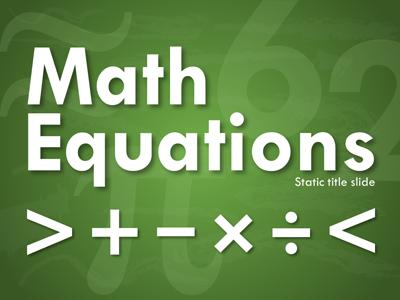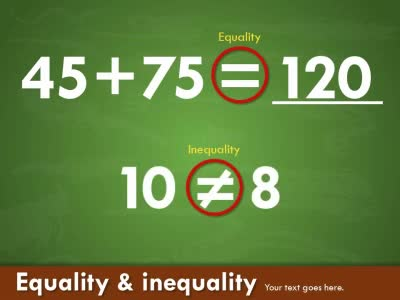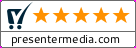# Math Equations

ITEM#: 16957
TYPE: PowerPoint Template

POWERPOINT
2007 - 2019
(PC & Mac)
KEYNOTE
(MAC)

There is also a WideScreen (16x9) version of this template available.

DESCRIPTION
This animated math equations themed template is an excellent way to present simple or complex math equations to your audience or classroom. You can use the provided symbols to create your own equations. Download for PowerPoint or Keynote.

KEYWORDS
math equations equation add subtract divide multiply pi 3.14 math mathematics geometry algebra square root exponent power equal greater than less than addition subtraction multiplication division equality angle right angle spherical angle measured angle degree arcminute arcsecond ray arc perpendicular parallel similarity equivalence equal by definition approximately equal approximately equal proportional to infinity symbol lemniscate approximation function of x school education teacher teach

# 8 Reasons To Subscribe

Limited Time Offer: Save up to 50% when you subscribe today!

SIMILAR IMAGES AND TEMPLATES (view all)
ID#3804 PRESENTATION CLIPART
Stick Figure Drawing Math Equation
ID#8047 VIDEO BACKGROUND
Rulers and Equations
ID#3747 PRESENTATION CLIPART
Mathematics Puzzle
ID#21026 PRESENTATION CLIPART
Chole Math Symbols Balance
ID#9088 PRESENTATION CLIPART
Math Text Symbols In Book
ID#16976 PRESENTATION CLIPART
Pi Sign
ID#3805 PRESENTATION CLIPART
Drawing E Equals MC Sqaured Math Equatio
ID#9019 VIDEO BACKGROUND
Math Concepts
ID#453 POWERPOINT ANIMATION
ID#12661 PRESENTATION CLIPART
Pi Symbol
ID#455 POWERPOINT ANIMATION
Math Symbols Bouncing Text Backdrop
ID#468 POWERPOINT ANIMATION
Jenny Holding Math Division Symbol
ID#477 POWERPOINT ANIMATION
Jenny Holding Math Subtraction Symbol
ID#460 POWERPOINT ANIMATION
Jenny Holding Math Multiplication Symbol
ID#458 POWERPOINT ANIMATION
Math Symbols Bouncing Up Down
ID#10061 PRESENTATION CLIPART
Brain Math Symbols
ID#12540 PRESENTATION CLIPART
Front Of Calculator

Your presentations are going to be amazing! | See Plans and Pricing
See Plans and Pricing© 2009-2020 Eclipse Digital Imaging, Inc.
Quality PowerPoint Templates and 3D Clipart